Ex 6.2

Chapter 6 Class 8 Squares and Square Roots
Serial order wise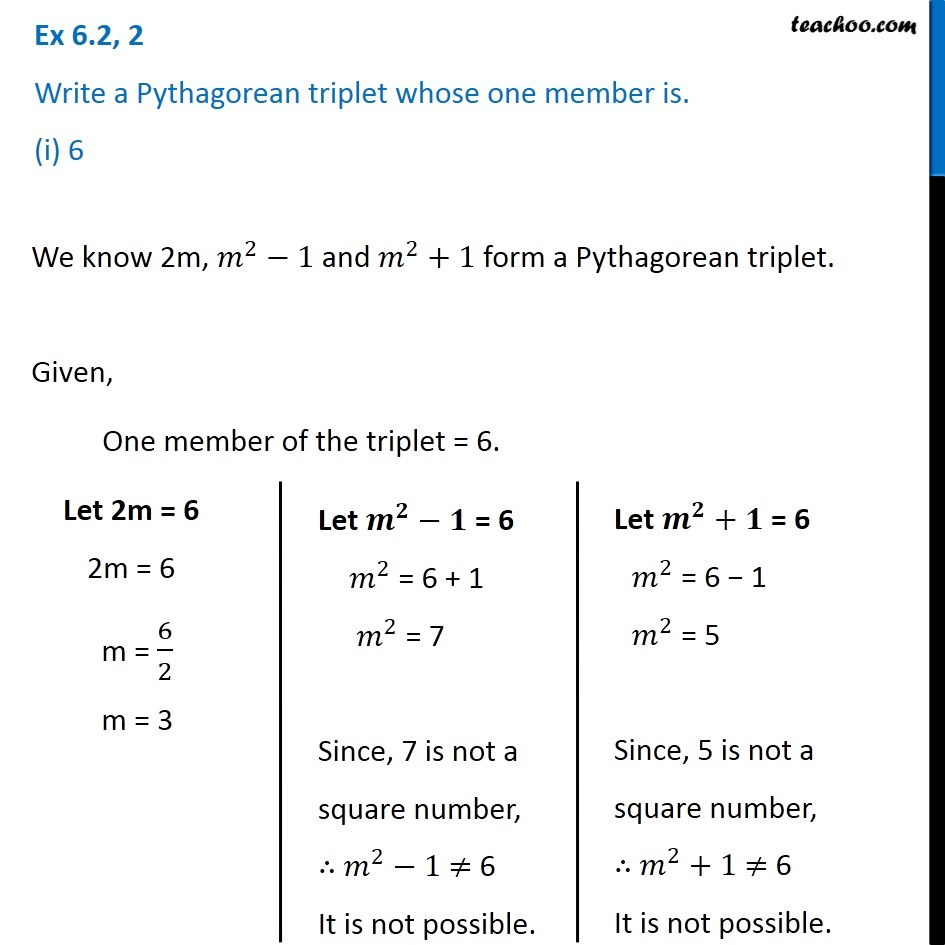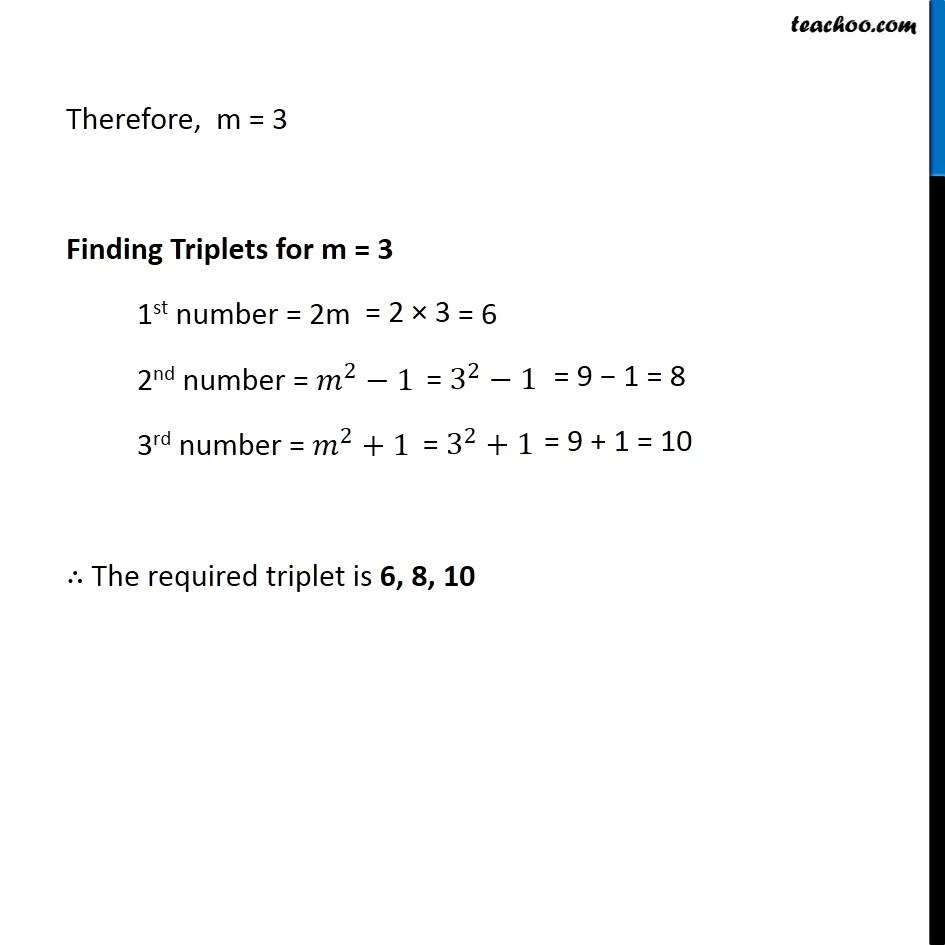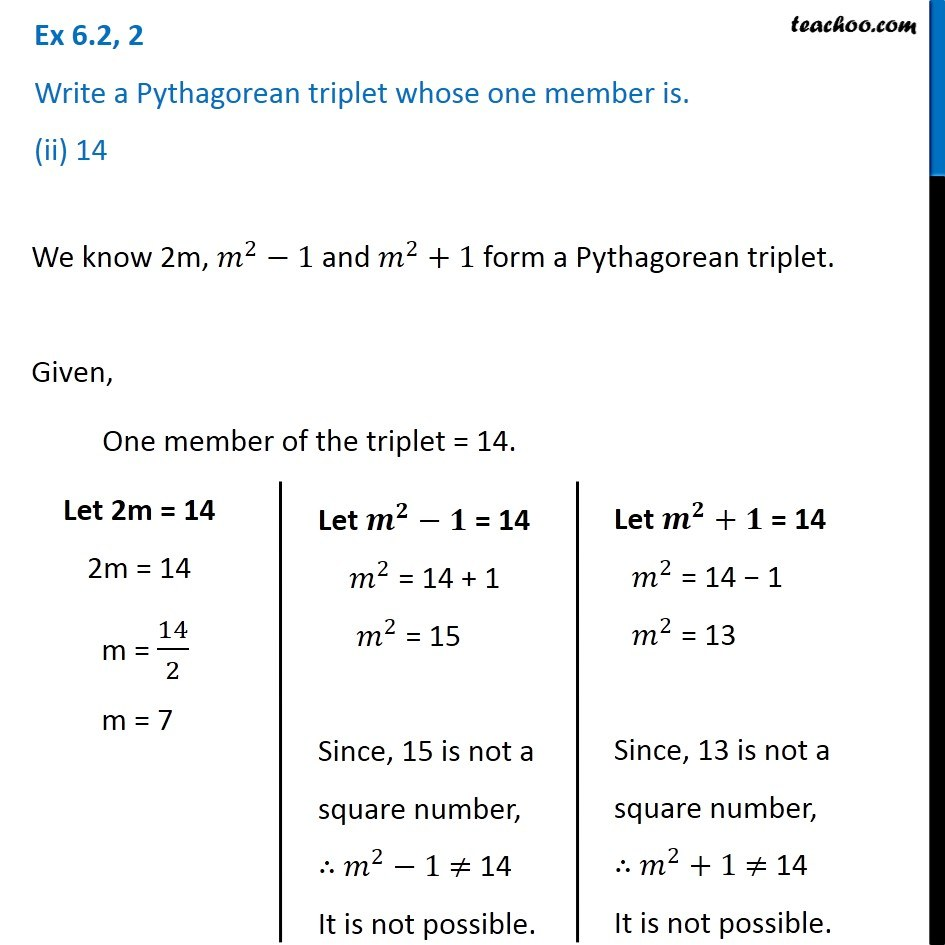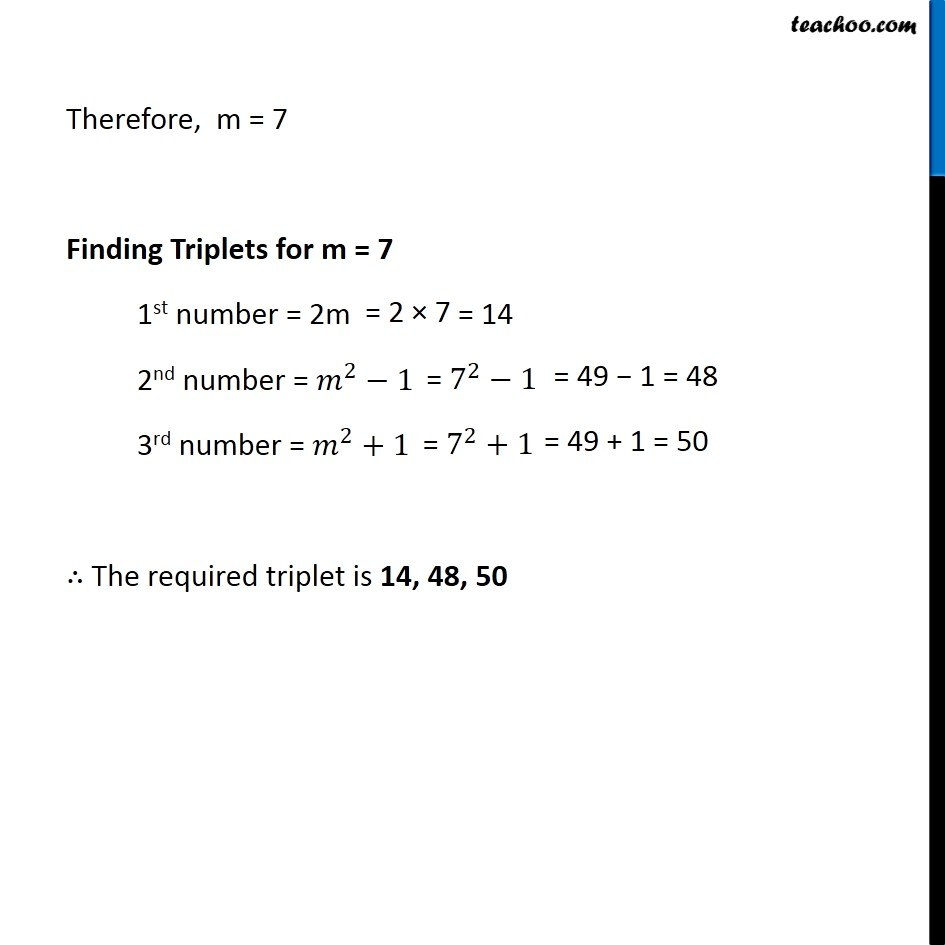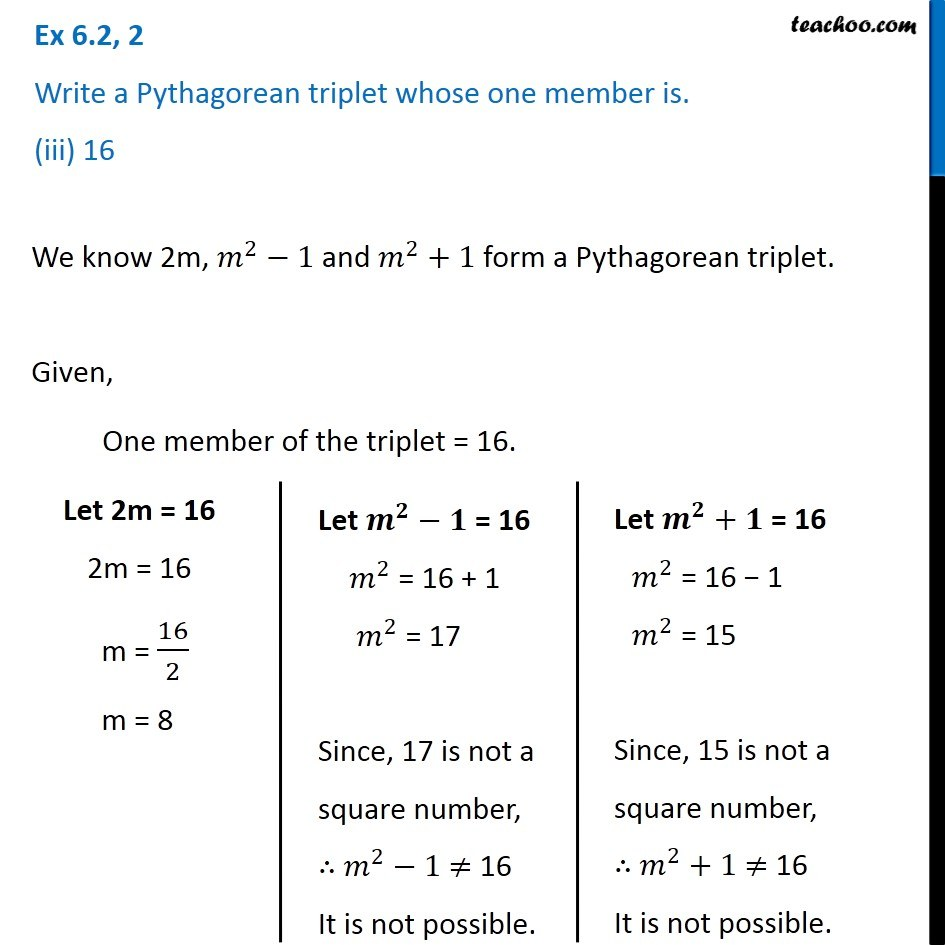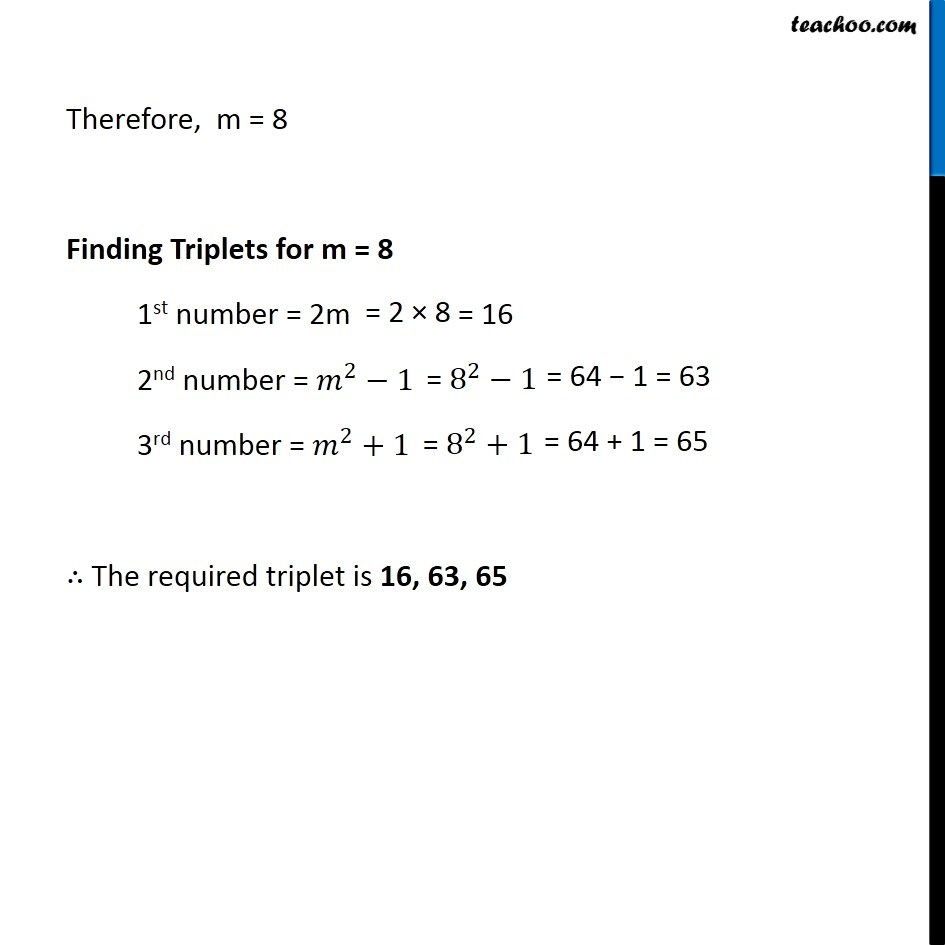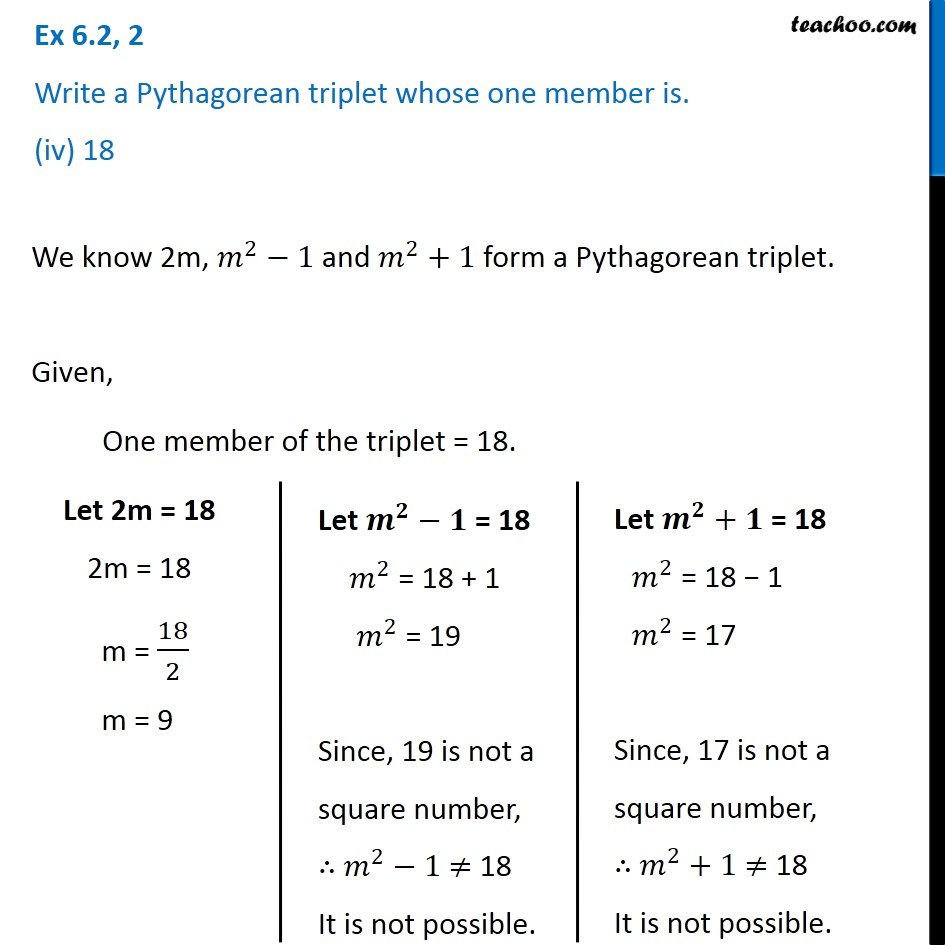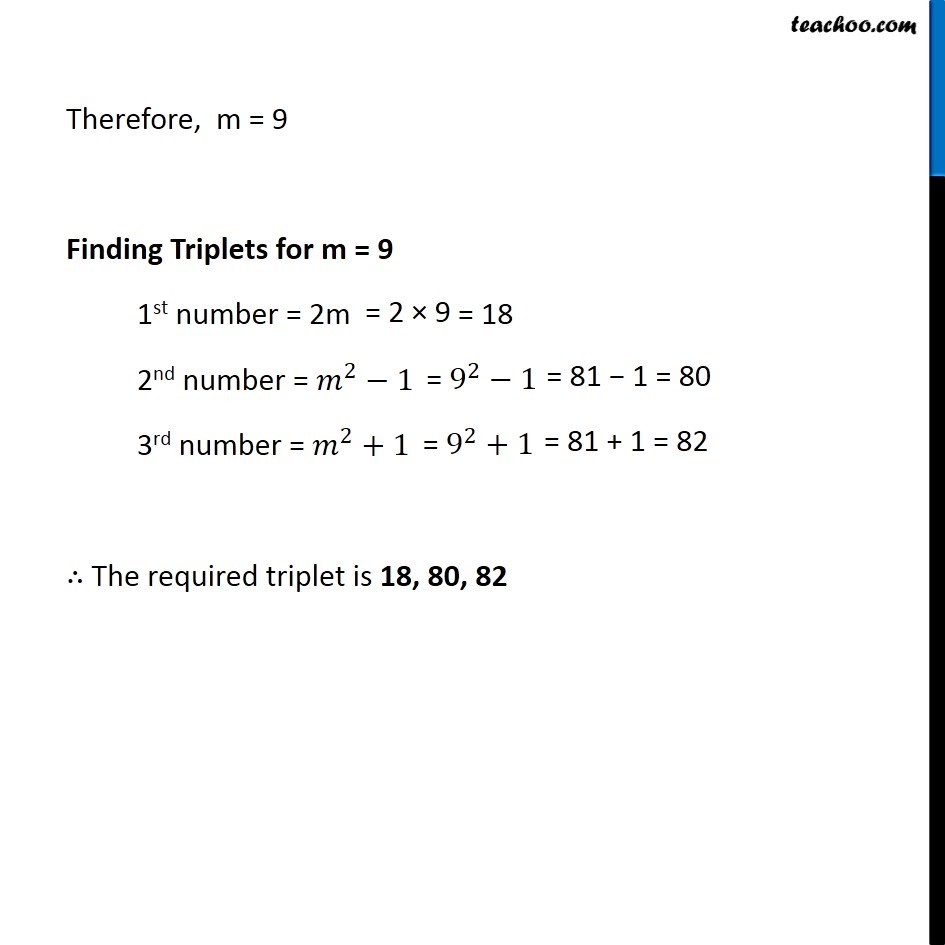### Transcript

Ex 6.2, 2 Write a Pythagorean triplet whose one member is. (i) 6We know 2m, 𝑚^2−1 and 𝑚^2+1 form a Pythagorean triplet. Given, One member of the triplet = 6. Let 2m = 6 2m = 6 m = 6/2 m = 3 Let 𝒎^𝟐−𝟏" = 6" 𝑚^2 = 6 + 1 𝑚^2 = 7 Since, 7 is not a square number, ∴ 𝑚^2−1 ≠ 6 It is not possible. Let 𝒎^𝟐+𝟏 = 6 𝑚^2 = 6 − 1 𝑚^2 = 5 Since, 5 is not a square number, ∴ 𝑚^2+1 ≠ 6 It is not possible. Therefore, m = 3 Finding Triplets for m = 3 1st number = 2m 2nd number = 𝑚^2−1 3rd number = 𝑚^2+1 ∴ The required triplet is 6, 8, 10 Ex 6.2, 2 Write a Pythagorean triplet whose one member is. (ii) 14We know 2m, 𝑚^2−1 and 𝑚^2+1 form a Pythagorean triplet. Given, One member of the triplet = 14. Let 2m = 14 2m = 14 m = 14/2 m = 7 Let 𝒎^𝟐−𝟏" = 14" 𝑚^2 = 14 + 1 𝑚^2 = 15 Since, 15 is not a square number, ∴ 𝑚^2−1 ≠ 14 It is not possible. Let 𝒎^𝟐+𝟏 = 14 𝑚^2 = 14 − 1 𝑚^2 = 13 Since, 13 is not a square number, ∴ 𝑚^2+1 ≠ 14 It is not possible. Therefore, m = 7 Finding Triplets for m = 7 1st number = 2m 2nd number = 𝑚^2−1 3rd number = 𝑚^2+1 ∴ The required triplet is 14, 48, 50 Ex 6.2, 2 Write a Pythagorean triplet whose one member is. (iii) 16We know 2m, 𝑚^2−1 and 𝑚^2+1 form a Pythagorean triplet. Given, One member of the triplet = 16. Let 2m = 16 2m = 16 m = 16/2 m = 8 Let 𝒎^𝟐−𝟏" = 16" 𝑚^2 = 16 + 1 𝑚^2 = 17 Since, 17 is not a square number, ∴ 𝑚^2−1 ≠ 16 It is not possible. Let 𝒎^𝟐+𝟏 = 16 𝑚^2 = 16 − 1 𝑚^2 = 15 Since, 15 is not a square number, ∴ 𝑚^2+1 ≠ 16 It is not possible. Therefore, m = 8 Finding Triplets for m = 8 1st number = 2m 2nd number = 𝑚^2−1 3rd number = 𝑚^2+1 ∴ The required triplet is 16, 63, 65 Therefore, m = 8 Finding Triplets for m = 8 1st number = 2m 2nd number = 𝑚^2−1 3rd number = 𝑚^2+1 ∴ The required triplet is 16, 63, 65 Ex 6.2, 2 Write a Pythagorean triplet whose one member is. (iv) 18We know 2m, 𝑚^2−1 and 𝑚^2+1 form a Pythagorean triplet. Given, One member of the triplet = 18. Let 2m = 18 2m = 18 m = 18/2 m = 9 Let 𝒎^𝟐−𝟏" = 18" 𝑚^2 = 18 + 1 𝑚^2 = 19 Since, 19 is not a square number, ∴ 𝑚^2−1 ≠ 18 It is not possible. Let 𝒎^𝟐+𝟏 = 18 𝑚^2 = 18 − 1 𝑚^2 = 17 Since, 17 is not a square number, ∴ 𝑚^2+1 ≠ 18 It is not possible. Therefore, m = 9 Finding Triplets for m = 9 1st number = 2m 2nd number = 𝑚^2−1 3rd number = 𝑚^2+1 ∴ The required triplet is 18, 80, 82